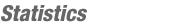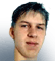JOINMatch Editorial
SRM 145
Tuesday, May 6, 2003

Match summary

Last night's problems were a good mix, with some dynamic programming, some simulation, and a couple relatively straightforward number problems. The set seemed to be pretty well-balanced also. Despite a rather wordy div 1 medium /div 2 hard problem, the submission percentages were all pretty well in line with the averages.

SnapDragon and Yarin were neck and neck during the challenge phase, and Yarin could have won the round if he had gotten one more challenge. But, in the end SnapDragon was able to hold on for the win, thanks to Yarin's resubmission of the 600 point problem. In division 2, shadowless was able to narrowly edge out first timer andlehay for the win.

# The Problems

DitherCounterUsed as: Division-II, Level 1:
 Value 250 Submission Rate 192 / 234 (82.05%) Success Rate 185 / 192 (96.35%) High Score pointone for 248.05 points

#### Implementation

Despite all of its fancy talk about bitmaps and dithering, this problem really has very little to do with any of that. To solve it, you simply have to go through each character in the given String[] (vector<string>) and see if that character is in dithered. Count how many such characters exist, and return that number.

Exercise MachineUsed as: Division-II, Level 2:
 Value 500 Submission Rate 154 / 234 (65.81%) Success Rate 119 / 154 (77.27%) High Score xxfobxx for 488.95 points

#### Implementation

This problem seems a little bit nonsensical at first glance. After all, what sort of exercise machine would be programmed in the manner described. However, the writer assured me that he has seen exercise machines that have wacky behavior along these lines.

So, to solve this, you can loop over either seconds, or over percentages. Either way, you can get one from the other, and then determine if they are both exact or not. Here is how you would do it if looping over percentages, if s is the total number of seconds that the workout lasts:

```   int ret = 0;
for(int i = 1; i<100; i++){
if((s*i)%100==0)ret++;
}
```
It also turns out that the answer is always gcd(s,100)-1. This is a lot harder to come up with, but much easier to code. I'll leave the proof as an exercise to the reader. As a hint of where to start, consider the case where gcd(s,100) = 2. In this case, the only number the display shows is 50%. Once you see this, it isn't too hard to work up to the general case.

VendingMachineUsed as: Division-II, Level 3:
 Value 1100 Submission Rate 18 / 234 (7.69%) Success Rate 10 / 18 (55.56%) High Score lgas for 570.28 points
Used as: Division-I, Level 2:
 Value 600 Submission Rate 84 / 127 (66.14%) Success Rate 73 / 84 (86.90%) High Score SnapDragon for 508.66points

#### Implementation

Despite the length of this problem, it is actually relatively simple to code. Basically, you just have to follow all of the instructions to the letter, and you will be fine.

The first thing to do is parse the prices input into some structure like a 2-D array. Then, since the purchases are given to you in order, it is pretty easy to apply them iteratively. For each purchase, if it is 5 or more minutes after the previous one, rotate to the most expensive column before applying the purchase. Then rotate to the column of the purchase item, and set t he price of the purchased item to 0. As you are buying items, if someone tries to buy an item whose price is 0, return -1. the rotations is relatively simple also. You don't actually need to rotate anything, just keep an int representing which column is facing out. Then, to get from column a to column b, you need either abs(a-b) seconds of rotation, or else numColumns - abs(a-b) seconds of rotation, whichever is less. Finding the most expensive column is also pretty straightforward. So, none of the components of this problem were really very difficulty, but putting things all together could be a little tricky, just because there are a lot of things going on. But basically, if you didn't have any bugs, there wasn't much here that was algorithmically difficult.

BonusesUsed as: Division-I, Level 1:
 Value 250 Submission Rate 126 / 127 (99.21%) Success Rate 112 / 126 (88.98%) High Score Yarin for 246.24 points

#### Implementation

Understanding how the division operator works is key to many problems, both in TopCoder and the real-world. In this problem we want to first find the percentage of the total point that each person has - truncated down to the nearest percentage. An easy way to do this after you've calculated the total number of points is percent[i] = (100 * points[i])/totalPoints. Then, you add up all the percentages, and find the number of percentage points that are left over, 100 - totalPercentage. Since the number of percentage points left over is relatively small, you can loop over them all and assign them one at a time, keeping track of who has already got one. One thing to note is that there will never be more percentage points left over than there are people. This is because when you truncate, you are removing less than one percentage point from each person. So, the total number of percentage points must be less than the number of people.

HillHikeUsed as: Division-I, Level 3:
 Value 1000 Submission Rate 21 / 127 (16.54%) Success Rate 13 / 21 (61.90%) High Score Yarin for 914.29 points

#### Implementation

This is a good dynamic programming problem, and can be solved with either a number of nested loops, or with memoized recursion. As with all DP problems, the trick is to come up with the correct recurrence. After that, it is relatively straightforward. So, the recursion:
There are quite a few variables that could potentially go into our recurrence. It turns out that the 4 essential ones are: current horizontal distance, current vertical height, number of landmarks that have been seen, and whether or not the maximum height has been reached yet or not. To ease the explanation, we will define a function f(h,d,lm,max), which represents the number of ways to reach distance d, height h, having seen lm landmarks, and having already reached the maximum height if and only if max is true

Now, the question is how to evaluate f(h,d,lm,max). Clearly, since the path goes strictly from lower horizontal distances to higher ones, f(h,d,lm,max) depends only on f(*,d-1,*,*), where *'s can represent any value. Also, since you can only move up one, down one, or stay at the same level, f(h,d,lm,max) = f(h-1,d-1,*,*) + f(h,d-1,*,*) + f(h+1,d-1,*,*). Now, there are two cases when considering whether or not the maximum height has been reached. If the height being considered, h, is the maximum height, then we have something like f(h,d,lm,true) = f(h-1,d-1,*,true) + f(h-1,d-1,*,false) + f(h,d-1,*,true), and f(h,d,lm,false) = 0. Otherwise, if h is not the maximum height, then f(h,d,lm,max) = f(h-1,d-1,*,max) + f(h,d-1,*,max) + f(h+1,d-1,*,max), for max either true or false.

Now, for the hard part: what to do about the landmarks. First we must observe that we should always assume that a landmark occurs as early as possible along a path. In other words, if considering whether or not a path is valid, a greedy approach to placing landmarks is always best. This means that, if we have placed lm landmarks, and the next landmark to be placed is at height h, then we can assume that the landmark was placed there, and move on to the next landmark. This allows us to finish our recurrence, with 4 cases:

• h = maxHeight, h = landmark[lm]:
f(h,d,lm,true) = f(h,d-1,lm-1,true) + f(h-1,d-1,lm-1,true) + f(h-1,d-1,lm-1,false)
f(h,d,lm,false) = 0
• h = maxHeight, h != landmark[lm]:
f(h,d,lm,true) = f(h,d-1,lm,true) + f(h-1,d-1,lm,true) + f(h-1,d-1,lm,false)
f(h,d,lm,false) = 0
• h != maxHeight, h = landmark[lm]:
f(h,d,lm,max) = f(h+1,d-1,lm-1,max) + f(h,d-1,lm-1,max) + f(h-1,d-1,lm-1,max)
for max = true of false
• h != maxHeight, h != landmark[lm]:
f(h,d,lm,max) = f(h+1,d-1,lm,max) + f(h,d-1,lm,max) + f(h-1,d-1,lm,max)
for max = true of false

Now, armed with this recurrence, we can implement it something like this (thanks to schveiguy for his pretty code). Note that while it is somewhat hidden, this code actually does implement the above recurrence:

```typedef long long int64;
struct HillHike
{
int64 numPaths(int distance, int maxHeight, vector<int> landmarks)
{
int64 cache1;
int64 cache2;
memset(cache1, 0, sizeof(cache1));
cache1 = 1;
landmarks.push_back(-1);
for(int i = 1; i < distance; i++){
memset(cache2, 0, sizeof(cache1));
for(int j = 1; j <= maxHeight; j++){
int ni = (j == maxHeight ? 1 : 0);
for(int k = 0; k < landmarks.size(); k++)
for(int d = -1; d <= 1; d++){
int lm = (j == landmarks[k] ? k + 1 : k);
cache2[ni][j][lm] += cache1[j + d][k];
cache2[j][lm] += cache1[j + d][k];
}
}
memcpy(cache1, cache2, sizeof(cache1));
}
return cache1[landmarks.size()-1];
}
};
```By lbackstrom
TopCoder Member